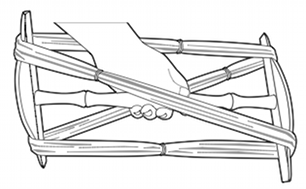# Spinning Frequently Asked Questions

Q How many yards are there in my skein?A Assuming a standard niddy noddy which is 15" long... when you've wound the yarn all the way around, that's four times 15 = 60 (that's how you get a 60" skein from a niddy-noddy which is 15" long).

So, take the skein and count how many full circles of yarn there are in it. Multiply that number by 60 to get the length in inches, and divide by 36 to turn that into yards.

Using a niddy noddy to wind a skein

<< Back to main FAQs list